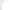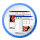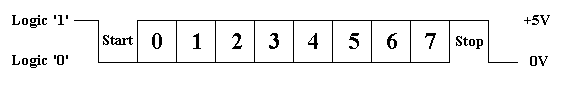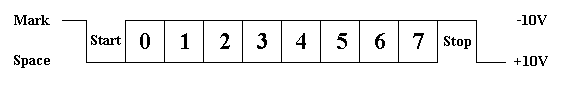Look RS232 > RS232C HardwareRS-232 WaveformsYet we have only spoken of asynchronous serial connection using RS-232. That is a clock signal is not sent with the data. Every word is synchronized using start bit and internal clock on either side keeps tabs on the timing.TTL/CMOS Serial Logic Waveform

The chart above shows the expected waveform from the UART when using the common 8N1 format. 8N1 signifies 8 Data bits, No Parity and 1 Stop Bit. The RS-232 line, when idle is in the Mark State (Logic 1). A transmission starts with a start bit which is (Logic 0). Then each bit is sent down the line, one by one. The LSB (Least Significant Bit) is sent first. A Stop Bit (Logic 1) is then added to the signal to make up the transmission.

The diagram shows the next bit after the Stop Bit to be Logic 0. This means another word is following, and this is its Start Bit. If there are no more data coming then the receive line will stay idle (logic 1). We have encountered something called a "Break" Signal. This is when the data line is held in a Logic 0 state for a time long enough to send an entire word. Therefore if you don't make the line idle again, then the receiving end will interpret this as a break signal.

The data sent using this method, is said to be framed. That is the data is framed between a Start and Stop Bit. If the Stop Bit is received as a Logic 0, then a framing error will occur. This is common, when both sides are communicating at different speeds.

The above diagram is only true for the signal immediately at the UART. RS-232 logic levels use +3 to +25 volts to signify a "Space" (Logic 0) and -3 to -25 volts for a "Mark" (logic 1). Any voltage between these regions (ie between +3 and -3 Volts) is undefined. Therefore this signal is put through a "RS-232 Level Converter". This is the signal present on the RS-232 Port of your computer, shown below.RS-232 Logic Waveform

The above waveform applies to the Transmission and Receiveing lines on the RS-232 port. These lines carry serial data, hence the name Serial Port. There are other lines on the RS-232 port which actually are Parallel lines. These lines (RTS, CTS, DCD, DSR, DTR, RTS and RI) are also at RS-232 Logic Levels.